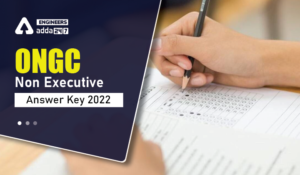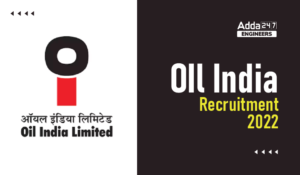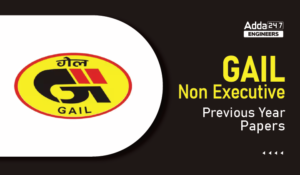Engineering Jobs   »   QUIZ : CIVIL ENGINEERING (09-09-2020)

# QUIZ : CIVIL ENGINEERING (09-09-2020)

Quiz: Civil Engineering
Exam: UPPSC
Topic: Miscellaneous

Each question carries 1 mark
Negative marking: 1/3 mark
Time: 15 Minutes

Q1. The vertical stress at depth Z directly below the point P is proportional to
(a) 1/z
(b) 1/z^2
(c) 1/z^3
(d) Z

Q2. The depth of tension crack in a soft clay is –
(a) 4c/r
(b) 2c/r
(c) C/2r
(d) C/rω

Q3. MPN index is a measure of
(a) 〖BOD〗_5
(b) Hardness
(c) Coliform bacteria
(d) Dissolved oxygen.

Q4. The difference between free water level in two wells is 4m and they are situated at 150 m apart an inert tracer takes 16 hrs to move from one well to other. If the porosity of aquifer is 30m. what is the coefficient of permeability of aquifer.
(a) 2.93 cm/sec
(b) 2 cm/sec
(c) 4 cm/sec
(d) 0.9 cm/sec

Q5. A waste water sample is diluted 50 times with fresh water the initial dissolve oxygen and after 5 days of incubation at 20 was found 17 mg/l and 7 mg/l the BOD of waste water sample is?
(a) 500 mg/l
(b) 1000 mg/l
(c) 100 mg/l
(d) 250 mg/l

Q6. The correct relationship between delta (∆) in cm, duty(D) in hectare per Cumec and base period (B) in days. Is
(a) ∆ =8.64 B/D
(b) ∆ =8.64 D/B
(c) ∆ =864 B/D
(d) ∆ =864 D/B

Q7. The super elevation needed for a vehicle travelling at a speed of 100 kmph on a horizontal curve of radios 250 m. on a road surface having coefficient of lateral friction of 0.15 is?
(a) 0.07
(b) 0.16
(c) 0.34
(d) 0.20

Q8. The penetration test for bitumen is conducted at a temperature of
(a) 100 °C
(b) 25°C
(c) 60 °c
(d) 40°c

Q9. A solid circular shaft having diameter of 100 mm is made up of a material which can withstand maximum shear stress of 110 MPa. The maximum torque (in kN) that the shaft can resist is
(a) 19.75
(b) 22.95
(c) 21.58
(d) 24.75

Q10. The BOD removal efficiency (in percentage) during primary treatment under normal condition is about
(a) 65%
(b) 85%
(c) 30%
(d) zero

Solutions

S1. Ans.(b)
Sol. As per Boussinesq
σ_Z=3/2 [1/(1+(r/Z)^2 )]^(5/2) P/Z^2
▭(σ_Z α 1/Z^2 )

S2. Ans.(b)
Sol. Active earth pressure in cohesive soil-
Pa =K_a rz-2C√(K_a )
Z=Zc=depth of tension crack
▭(P_a=0)
0=K_a rZ_C-2C√(K_a )
▭(Z_C=2C/√(K_a ))
for cohessive soil (ϕ=0) (K_a=1)
▭(Z_C=2C/r)

S3. Ans.(c)
Sol. MPN (most probable number)
It is a measure of coliform bacteria. Membrane filter technique & coliform index test also used in MPN test.

S4. Ans.(a)
Sol. Seepage velocity (V_S )=(discharge velocity (V))/(Porosity (n) )
Distance/Time=V/n
150/16=V/0.30
▭(V=2.8125 m\/hr)
V=K.i
2.8125=K×(∆h/L)
2.8125=K×4/150
K=105.46 m\/hr
▭(K=2.93 cm\/sec)

S5. Ans.(a)
Sol. BOD = (DO_i-DO_f )×Dilution factor
=(17-7)×50
=500 mg\/l

S6. Ans.(c)
Sol. ∆ =8.64 B/D (m)
∆ =864 B/D (cm)

S7. Ans.(b)
Sol. e+f=V^2/127R
e+0.15=(100)^2/(127×250)
▭(e=0.165)

S8. Ans.(b)
Sol. The penetration test for bitumen is conducted at 25°C

S9. Ans.(c)
Sol. τ_max=16T/(πD^3 )
T=(πD^3)/16 τ_max
=(π (100)^3)/16×110
=21.5875 .kN-m.

S10. Ans.(c)
Sol. In the primary treatment BOD removal efficiency = 30 – 35%
Suspended solid removal efficiency = 60 – 7-%

Sharing is caring!

Thank You, Your details have been submitted we will get back to you.
•DVC Interview Schedule 2022, Download DV...
•ONGC Non Executive Answer Key 2022, Down...
•Oil India Recruitment 2022, Apply Online...
•GAIL Non Executive Previous Year Papers,...
•GAIL Non Executive Previous Year Cutoff ...
•APWD Previous Year Papers, Check here fo...